# Playing with Quantifier Satisfaction

 Nikolaj Bjørner and Mikoláš Janota Microsoft Research nbjorner@microsoft.com, mikolas.janota@gmail.com

## Quantified Formulae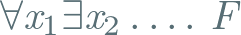• a game between universal and existential player
• E.g.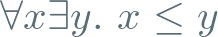, winning strategy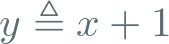### Solving QBF (QESTO)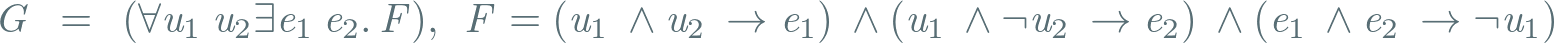•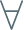: starts.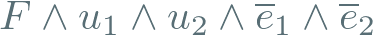is false.
•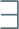: strikes back.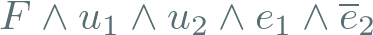is true.
•: has to backtrack.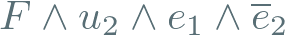is already unsat.
•: learns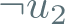.
•: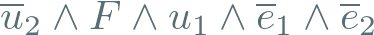is false.
•: counters -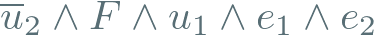is true.
•: has yet again to consider what went wrong by determining the core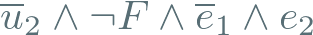, which is empty. The universal player has to give up and the existential player established that the formula is true.

### Model-based Projections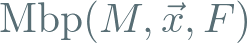•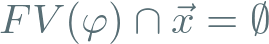,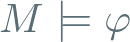, and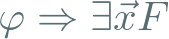.
• The size of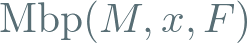is no larger than the size of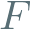.
• There is a sequence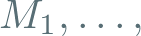of models of, such that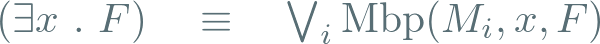.
• Let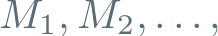be an infinite sequence of models of literals, then there is a finite set of equivalent projections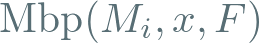.

### Model-based Projection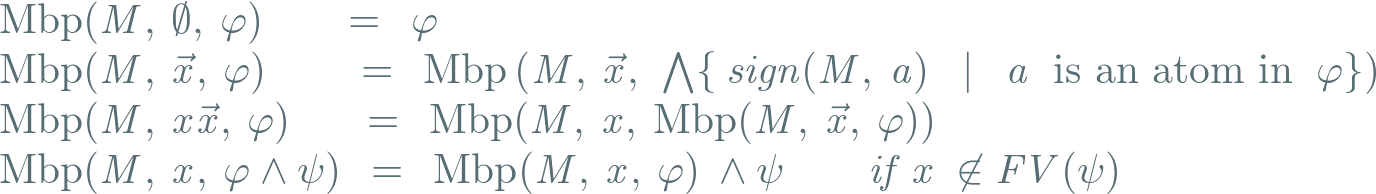def sign(M,a): if M(a) return a else return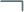a

### Model-based Projection for LRA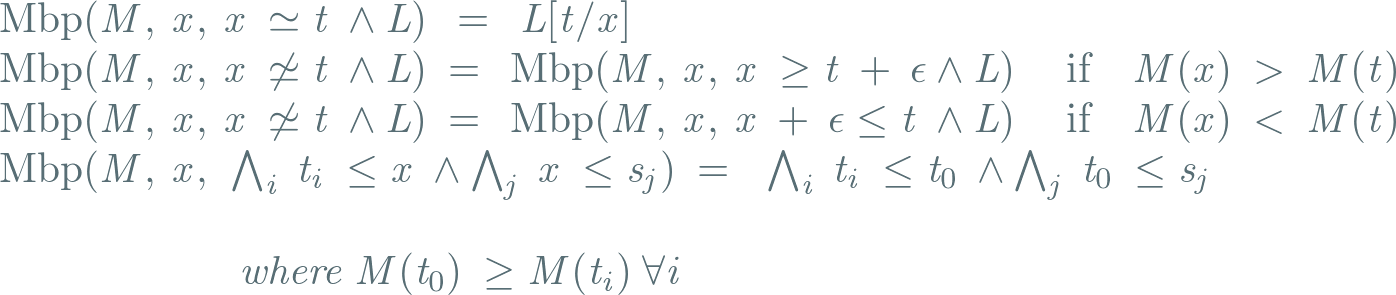### Initialization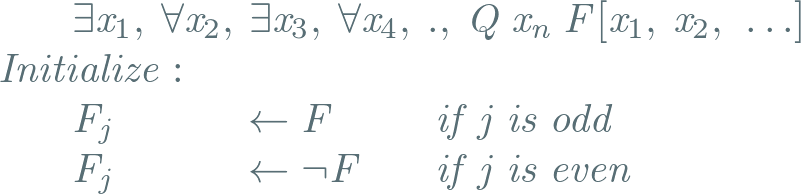def level(j,a): return max level of bound variable in atom a of parity j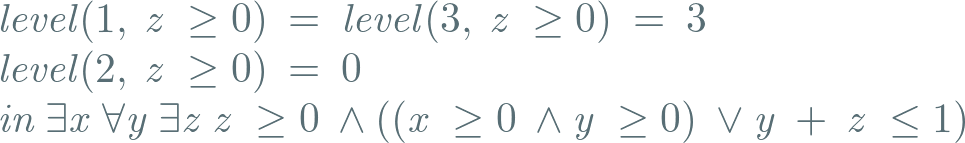### Property Directed QSAT Algorithm

  def strategy(M,j): return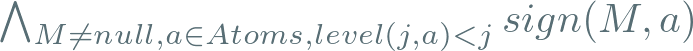def tailv(j): return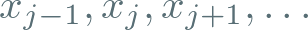j = 1  M = null  while True:    if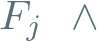strategy(M, j) is unsat:      if j == 1:         return F is unsat      if j == 2:        return F is sat      C = Core(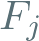, strategy(M, j))      J = Mbp(tailv(j), C)      j = index of max variable in J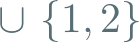of same parity as j=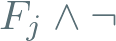J      M = null    else:      M = current model      j = j + 1          

### Proof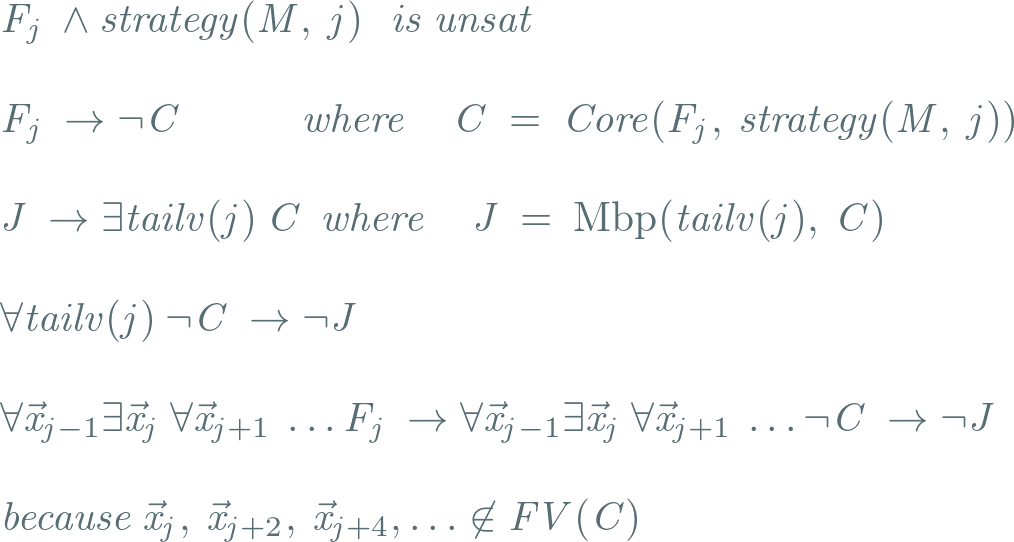Note: core foris implicant of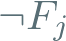.

### On Quantifier Elimination

• Extends to Quantifier Elimination.

• Enumerate literals that imply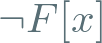• by tracking clauses asserted at level 2.

### On Strategies

• Our base strategy uses limited look-ahead.

• It is possible to formulate stronger strategies (based on models)

### Some Experiments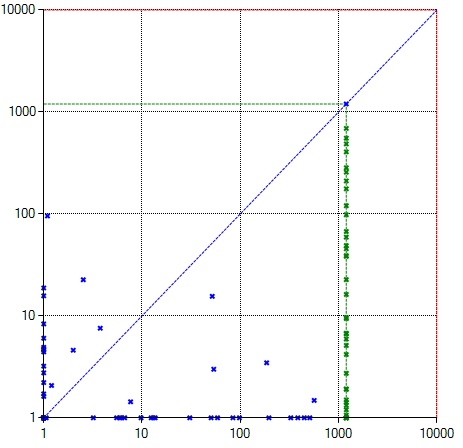Figure 1. LRA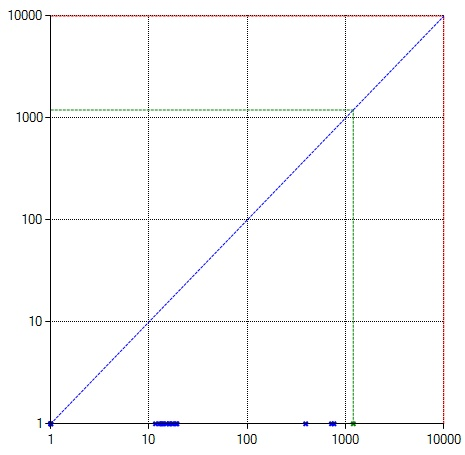Figure 2. LIA

### Summary and Future Work

• Solving quantified theories.
• Using Model-based projections and cores.
• Symmetric view onand.
• More theories?
• More advances lookahead? (hints in afternoon talk)